Class 10 RD Sharma Solutions – Chapter 12 Some Applications of Trigonometry – Exercise 12.1 | Set 3

• Last Updated : 28 May, 2021

Question 53. From the top of a building AB, 60 m high, the angles of depression of the top and bottom of a vertical lamp post CD are observed to be 30° and 60° respectively. Find

(i) the horizontal distance between AB and CD.

(ii) the height of the lamp post

(iii) the difference between the heights of the building and the lamp post.

Solution:

Let us considered AB is the building and CD is the vertical lamp

From point A, the top of the building angles of depression of C and D are 30° and 60°

Height of building AB = 60 m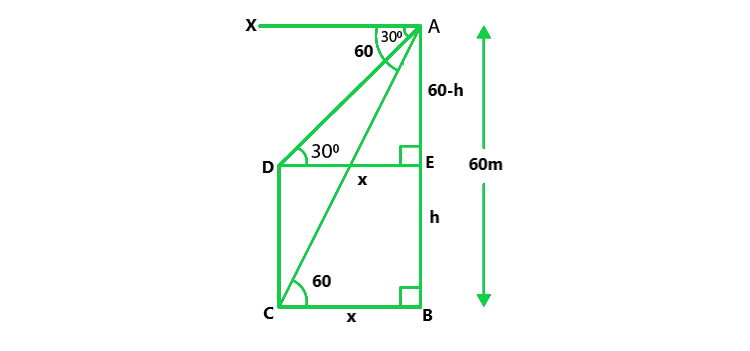Let us assume that the height of CD = h

Draw CE || DB || AX

∴ ∠ACE = ∠XAC = 30° and ∠ADB = ∠XAD = 60°

EB = CD = h and AE = 60 – h

Let DB = CE = x

Now in ΔACE,

tan 30° = (60 – h)/x

1/√3 = (60 – h) / x

x = √3 (60 – h)        …….(i)

tan 60° = AB/DB = √3 = 60/x

x = 60/√3 = 34.64 m

Hence, 20√3 = √3(60 – h)

h = 40 m

Hence, the height of vertical lamp is 40 m.

(i) Horizontal distance b/w them = DB = x

20√3 m = 3464 m

(ii) Height of vertical lamp = 40 m.

(iii) Difference b/w the heights = 60-40 = 20 m.

Question 54. Two boats approach a lighthouse in mid­sea from opposite directions. The angles of elevation of the top of the lighthouse from two boats are 30° and 45° respectively. If the distance between the two boats is 100 m, find the height of the lighthouse.

Solution:

Let us considered LH is the lighthouse and

A and B are two boats on the opposite directions of the lighthouse

which are making the angle of elevation of the top L of the lighthouse as 30° and 45°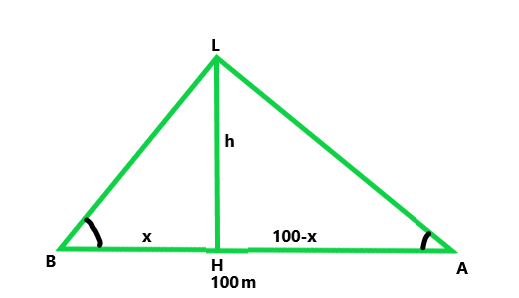Given that, AB = 100m

Let us considered LH is h and BH = x,

HA = 100 – x

Now in △LAH,

tan 30° = LH/ HA = h/(100 – x) = 1/√3 = h/(100 – x)

x = 100 – √3h           …….(i)

Similarly, in △LHB,

tan 45° = LH/BH = 1 = h/x

h = x  ……(ii)

From eq(i) and (ii), we get

h = 100 – √3h

h + √3h = 100

h(√3+1) = 100

h = 100/(√3+1)

On rationalize above term and we get,

h = 50(√3 – 1)

Hence, the height of the lighthouse is 50(√3 – 1) m.

Question 55. The angle of elevation of the top of a hill at the foot of a tower is 60° and the angle of elevation of the top of the tower from the foot of the hill is 30°. If the tower is 50 m high, what is the height of the hill?

Solution:

Let us considered TR be the tower, HL is the hill and

angles of elevation of the top of the hill are 60° and top of the tower is 30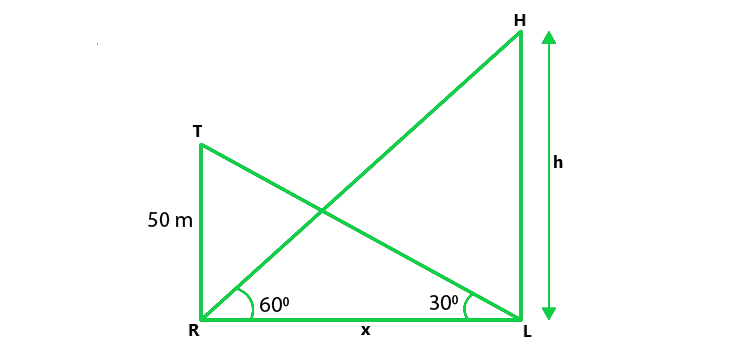Given, TR = 50 m

Let us considered the height of hill HL = h

and RL = x

Now in △TRL,

tan 30° = TR/RL = 50/x = 1/√3

x = 50√3         …….(i)

Similarly, in △HRL,

tan 60° = HL/RL = h/x

√3 = h/50√3

h = 50√3 x √3 = 150 m

Hence, the height of the hill is 150 m.

Question 56. A moving boat is observed from the top of a 150 m high cliff moving away from the cliff. The angle of depression of the boat changes from 60° to 45° in 2 minutes. Find the speed of the boat in m/h.

Solution:

Let us considered the distance BC be x m and CD be y m.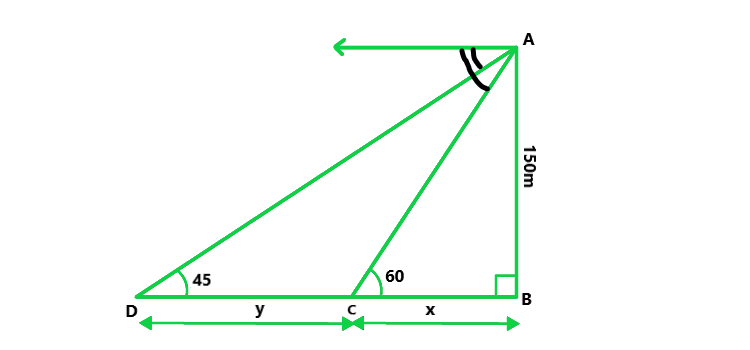In △ABC,

tan 60° = AB/BC = 150/x

√3 = 150/x

x = 150/√3      …….(i)

In △ABD,

tan 45° = AB/BD = 150 / (x + y)

x + y = 150

y = 150 – x

Now, using eq(i) we get,

y = 150 – 150/√3 = 150(√3 – 1) / √3

Time is taken to move from point C to point D in 2 min = 2/60 = 1/30 h

Now, Speed = Distance / Time = y / (1/30)

(150(√3-1) / √3) / (1/√3) = 1902 m/h

Question 57. From the fop of a 120 m high tower, a man observes two cars on the opposite sides of the tower and in a straight line with the base of the tower with angles of depression as 60° and 45°. Find the distance between the cars. (Take √3  = 1.732)

Solution:

Let us considered BD be the tower and A and C be the two points on the ground.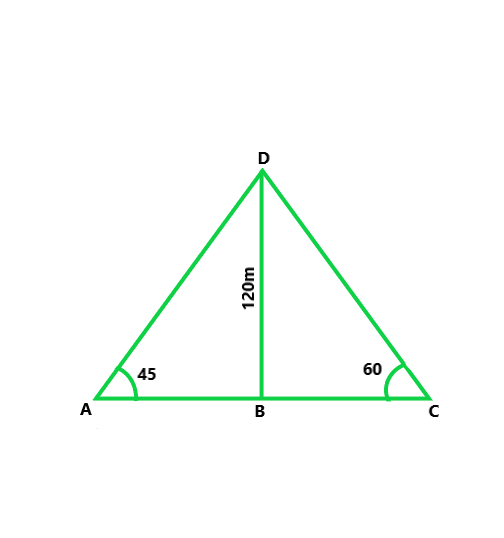Given that, BD, the height of the tower = 120m

∠BAD = 45°, ∠BCD = 60°

tan 45° = BD/BA

1 = 120 / BA

BA = 120 m              ————–(i)

tan 60° = BD / BC

√3 = 120/BC

BC = 120/√3 = 120√3 / 3 = 40√3 = 69.28 m        —————-(ii)

Distance b/w the two points A and C

AC = BA + BC

120 + 69.28 = 189.28 m

Question 58. Two points A and B are on the same side of a tower and in the same straight line with its base. The angles of depression of these points from the top of the tower are 60° and 45° respectively. If the height of the tower is 15 m, then find the distance between these points.

Solution:

Let us considered TR be the tower and

A, B are two objects which make angles of elevation with the top of the tower as 45° and 60°.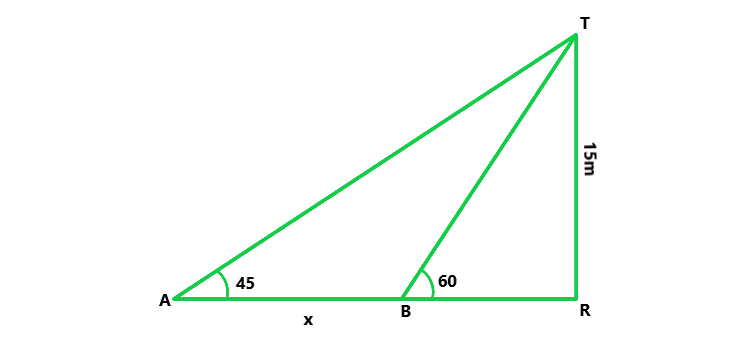Given that, the height of Tower TR = 15 m

Let us considered, the distance b/w B and A = x m

Now in △TAR,

tan 45° = 15/AR

1 = 15/AR

AR = 15

Similarly, in △TBR,

tan 60° = TR/BR = 15/BR

√3 = 15/BR = BR = 15/√3

Now AB = x = AR – BR,

15 – 15/√3 = 15 – 15 x 1.732 = 6.34 m

So, the distance b/w A and B = 6.34 m.

√3/2 = 12.7/BP = BP = 12.7 x 2/√3

BP = 14.68 km

Hence, P fire station is nearer and its team will reach the building after coming 14.6 km.

Question 59. A fire in a building B is reported on the telephone to two fire stations P and Q, 20 km apart from each other on a straight road. P observes that the fire is at an angle of 60° to the road and Q observes that it is at an angle of 45° to the road. Which station should send its team and how much will this team have to travel?

Solution:

Let us considered B is the building one fire and P and Q the fire stations which are 20 km apart i.e., PQ = 20 km.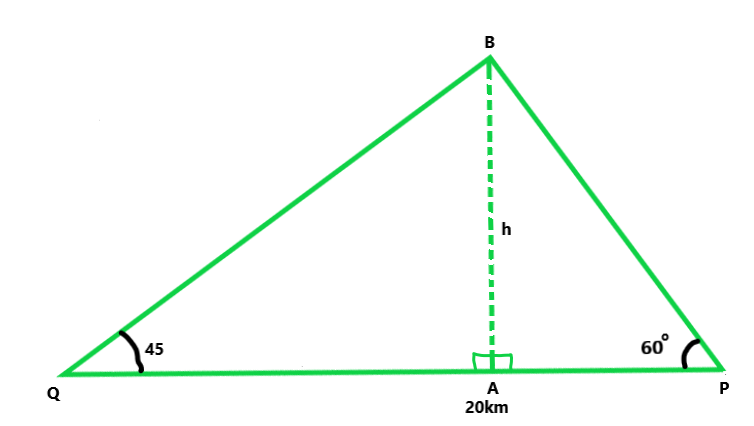Given that, P and Q observes the angles with B, as 60° and 45°

Now, draw BA ⊥ PQ

Let AB = h Now in right ΔBAQ,

tan 45° = h/AQ

1 = h/AQ = AQ = h

Hence, PA = 20 – h

Similarly, in △BAP,

tan 60° = BA/Ap

√3 = h / (20 – h)

h(√3 + 1) = 20√3

On rationalize above term and we get,

h = 20√3(√3 – 1) / 2 = 12.7 km

Hence, AQ = 12.7 km and AP = 20 – 12.7 = 7.3 km

Now sin 45° = P/H

1/√2 = h / BQ

BQ = 12.7 x √2 = 12.7 x 1041

BQ = 17.91 km

Similarly, sin 60° = BA/BP = 12.7 / BP

BP = 12.7 x 2 / √3

BP = 14.68 km.

Hence, P fire station is nearer and its team will reach the building after coming 14.6 km.

Question 60. A man on the deck of a ship is 10 m above the water level. He observes that the angle of elevation of the top of a cliff is 45° and the angle of depression of the base is 30°. Calculate the distance of the cliff from the ship and the height of the cliff.

Solution:

Let us assume M is a man on the deck MN such that MN = 10 m, AB is the cliff

So, from M the angle of elevation of A is 45°

and angle of depression of B is 30°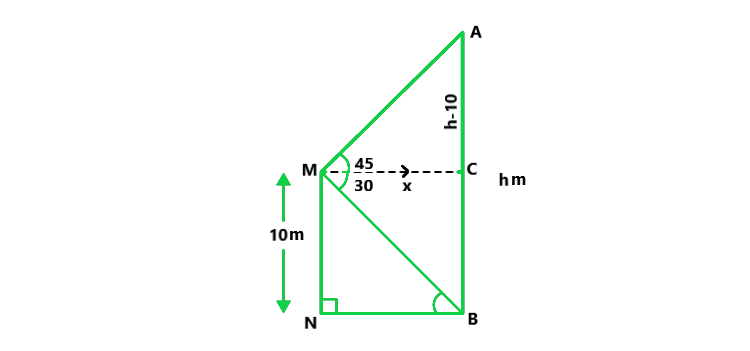Now, draw MC || NB

Hence ∠MBN = ∠CMB

Let us considered AB = h, NB = MC = x and AC = h – 10

Now in △AMC,

tan 45° = (h – 10) / x

1 = (h – 10) / x

x = h – 10        …….(i)

Similarly, in △MBN,

tan 30° = MN/BN

1/√3 = 10/x

x = 10√3   ……(ii)

From eq(i) and (ii), we get

h – 10 = 10√3

h = 10 + 10√3 = 27.32

and x = h – 10 = 27.32 – 10 = 17.32

Hence, the height of the cliff is 27.32 m and the distance of the ship from the cliff is 17.32 m.

Question 61. A man standing on the deck of a ship, which is 8 m above water level. He observes the angle of elevation of the top of a hill as 60° and the angle of depression of the base of the hill as 30°. Calculate the distance of the hill from the ship and the height of the hill.

Solution:

Let us assume M is the man on the deck MN such that MN = 8 m and AB is the hill

Now, from M, the angle of elevation of A is 60° and angle of depression of B is 30°.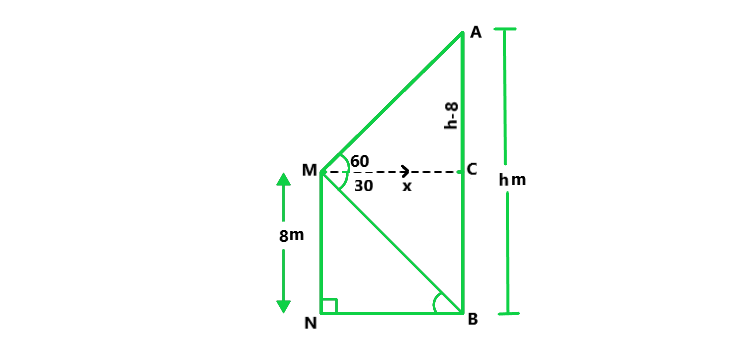Draw MC || NB

Hence, ∠MBN = ∠CMB

Let us assume AB = h, NB = MC = x

AC = h – 8

Now in △AMC,

tan 60° = AC/MC = h – 8 / x = √3

x = h – 8 / √3 …….(i)

Similarly, in △MBN,

tan 30° = MN/NB = 8/x

1/√3 = 8/x

x = 8√3    …….(ii)

From eq(i) and (ii), we get

8√3 = (h – 8)/√3

h = 32

and x = 8√3 or 13.858 m

Hence, the height of the hill = 32 m and the distance between ships = 13.858 m.

Question 62. There are two temples, one on each bank of a river, just opposite to each other. One temple is 50 m high. From the top of this temple, the angles of depression of the top and the foot of the other temple are 30° and 60° respectively. Find the width of the river and the height of the other temple.

Solution:

Let us assume AB and CD are two temples on the banks of the river.

Given that, AB = 50 m

Now, from A, the angles of depression of the top and bottom of the other temple are 30° and 60°.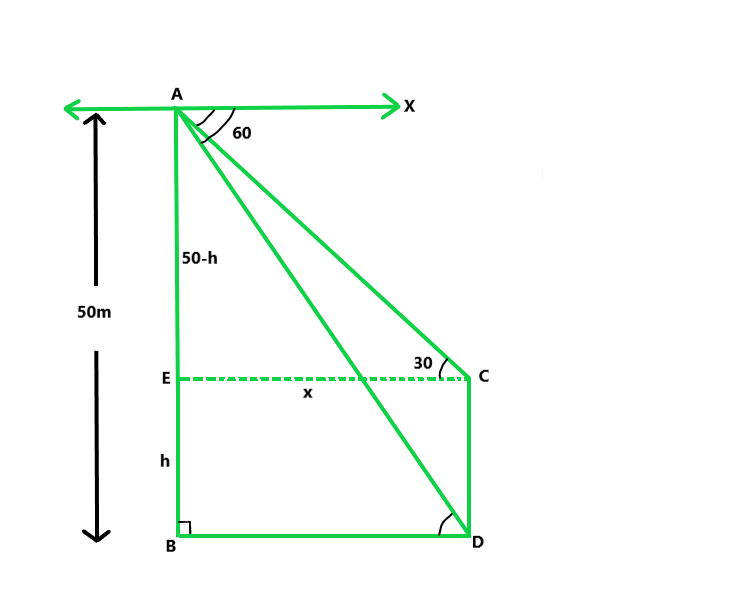Let us assume CD = h

So that AE = 50 – h, let BD = EC = x

Now in △ABD,

tan 60° = 50/x = √3 = 50/x

x = 50√3/3     …….(i)

Similarly, in △AEC,

tan 30° = AE/EC = (50 – h)/x

1/√3 = (50 – h)/x

x = √3(50 – h)            …….(ii)

From eq(i) and (ii), we get

50√3/3 = √3(50 – h)

h = 33.33 m

Hence, the height of the second temple is 33.33 m and the width of the river = 50√3/3 = 28.23 m.

Question 63. The angle of elevation of an aeroplane from a point on the ground is 45°. After a flight of 15 seconds, the elevation changes to 30°. If the aeroplane is flying at a height of 3000 meters, find the speed of the aeroplane.

Solution:

Let us assume A is the plane flying in the sky at its height of 3000 m i.e., AB = 3000 m

Now, P is a point on the ground which from an angle of elevation of 45° at A and

then after a flight of 15 seconds at A’ the angle of elevation because 30°.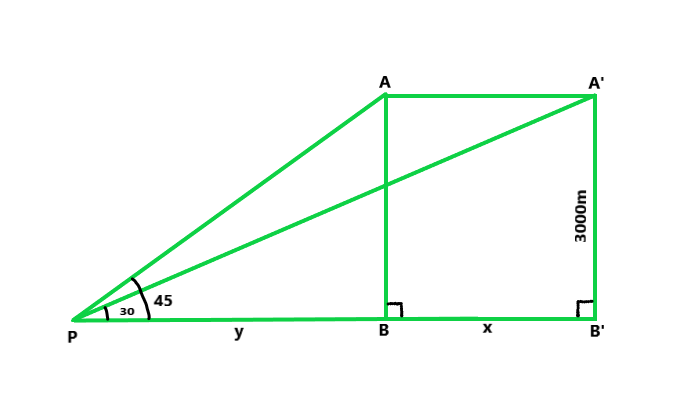Let us assume PB = y and BB’ = x m

Now in △APB,

tan 45° = AB/PB = 3000/y

y = 3000 m.           …….(i)

Similarly, in △A’PB’

tan 30° = A’B’/PB’ = 1/√3 = 3000/(x + y)

x + y = 3000√3

x = 3000√3 – y

x = 3000(√3 – 1)

= 2196 m.

Hence the distance of 2196 m is covered in 15 seconds.

Speed of the plane = (2196x60x60)/15 = 527040 m/hr = 527.04 km/hr

Question 64. An aeroplane flying horizontally 1 km above the ground is observed at an elevation of 60°. After 10 seconds, its elevation is observed to be 30°. Find the speed of the aeroplane in km/hr.

Solution:

Let us considered A be the aeroplane and AB is the height which 1 km and

makes an angle of elevations of 60° from a point P on the ground.

Now, after moving 10 second’s flight, the angle of elevation becomes 30° from P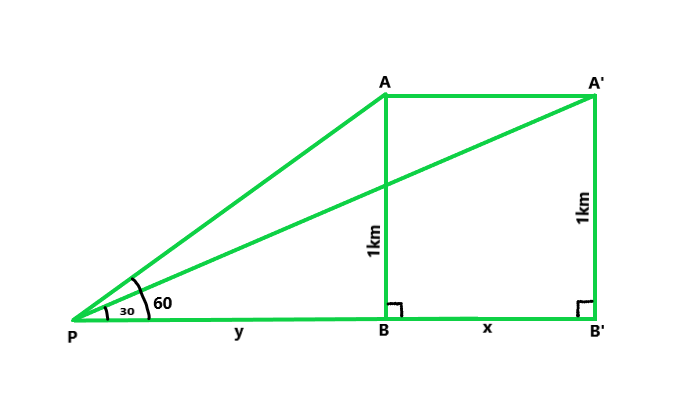Giventhat, A’B’ = AB = 1 km = 1000 m

Let us assume PB = y and BB’ = x

Now in ΔAPB,

tan 60° = AB/PB = 1000/y = √3

y = 1000/√3            …….(i)

Similarly, in △A’PB’,

sin 30° = A’B’/PB’ = 1/√3 = 1000/(x + y)

x + y = 1000√3

x = 1000(√3 – 1)

= 1154.73 m

Now 1154.73 m is covered in 10 seconds.

Hence, speed per hour = (1154.73 x 60 x 60)/10 x 1000 = 415.7 km/hr.

Question 65. A tree standing on a horizontal plane is leaning towards the east. At two points situated at a distance a and b exactly due west on it, the angles of elevation of the top are respectively a and p. Prove that the height of the top from the ground is ((b – a) tanα tanβ) / (tanα – tanβ).

Solution:

Let us considered CD is the tree which is leaning towards the east and

A and B are two points on the West making angles of elevation with top C of the tree as α and β

A and B are at the distance of a and b from the foot of the tree CD, then AD = a, BD = b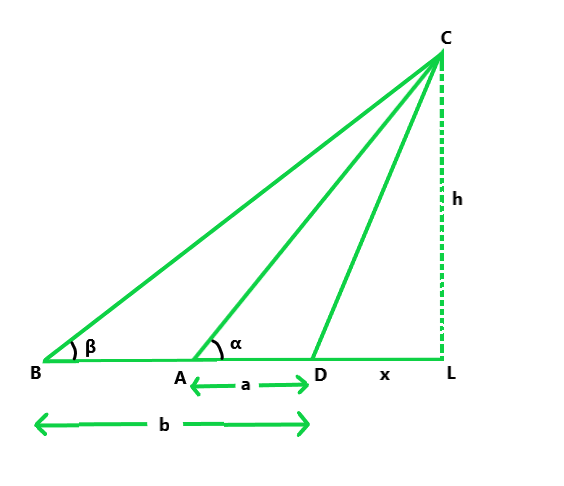Now, draw CL ⊥ BD produce and let DL = x and CL = h

So, in △ CAL,

tan α = h/(a + x)

a + x = h/tanα       …..(i)

Similarly, in △CBL,

tan β = CL/BL = h/(b + x) = h/tanβ      …..(ii)

From eq(i) and (ii), we get

x = h/tanα – α

x = h/tanβ – β

h/tanβ – β = h/tanα – α

h(1/tabβ – 1/tanα) = b – a

h = (β – α) tanα tanβ / (tanα – tanβ)

Hence proved!

Question 66. The angle of elevation of a stationery cloud from a point 2500 m above a lake is 15° and the angle of depression of its reflection in the lake is 45°. What is the height of the cloud above the lake level? (Use tan 15° = 0.268)

Solution:

Let us considered C is the cloud over a lake LK

Now, from a point, M which, is 2500 m above the lake level,

the angle of elevation of C is 15° and

the angle of depression of the reflection of C in the lake which is R is 45°.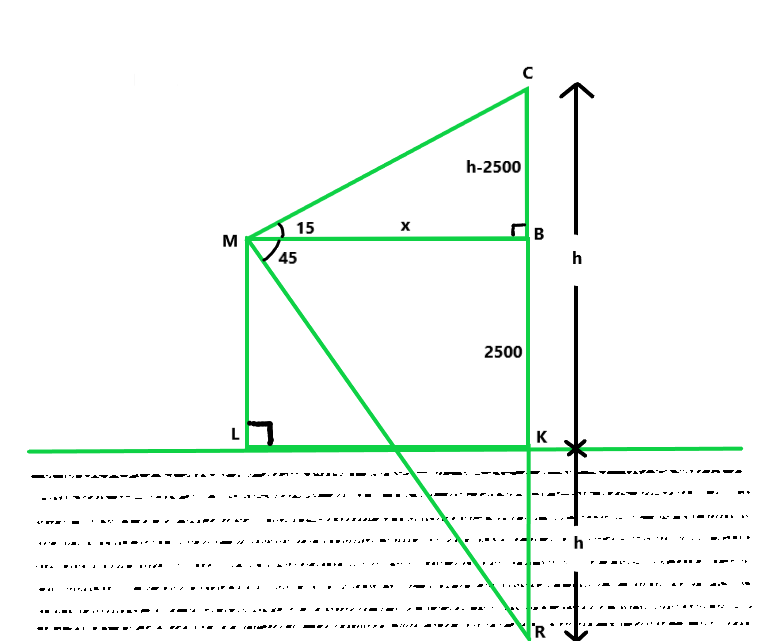Let us assume CK = h then the reflection KR = h

BK = ML = 2500 m then CB = h – 2500

Let MB = LK = x

Now in △CMB,

tan 15° = (h – 2500)/x = x = (h – 2500)/tan 15         …..(i)

Similarly, in △BMR,

tan 45° = BR/MB

1 = (h + 2500) / x

x = h + 2500   ……(ii)

From eq(i) and (ii), we get

h + 2500 = (h – 2500)/tan15

h + 2500 = (h – 2500)/0.2679

h = 4329.67 m

Hence, the height of the cloud is 4329.67 m.

Question 67. If the angle of elevation of a cloud from a point h meters above a lake is a and the angle of depression of its reflection in the lake be p, prove that the distance of the cloud from the point of 2hsecα/tanβ − tanα.

Solution:

Let us considered C be the cloud and from a point, M which h m is above the lake level

angle of elevation is α and angle of reflection of the cloud C, is β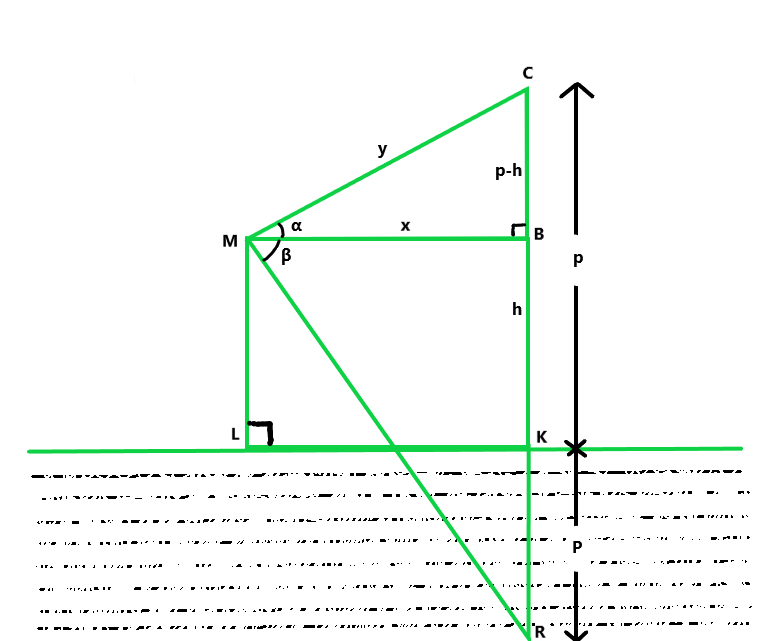Let LK = MN = x

MC = y, height of cloud CK = p

TK = h, CN = p – h and KR = p

Now in △CMN,

cosα = MN/CM = x/y

x = y cosα   ……(i)

and tanα = (p – h)/x = p – h = x tanα

p = h + x tanα           ……(ii)

Similarly, in △MNR,

tanβ = NP/MN = (p + h)/x

p + h = x tanβ     ……(iii)

From eq(ii) and (iii), we get

h + x tanα + h = x tanβ

x = 2h/(tanβ – tanα)

y cosα = 2h/(tanβ – tanα)

y = 2h secα / (tanβ – tanα)

Hence, distance of cloud from point = 2h secα / (tanβ – tanα)

Question 68. From an aeroplane vertically above a straight horizontal road, the angles of depression of two consecutive milestones on opposite sides of the aeroplane are observed to be a and p. Show that the height in miles of the aeroplane above the road is given by tanα tanβ / tanα + tanβ.

Solution:

Let us considered A is aeroplane and C and D are two such points that the

angles of depression from A are α and β respectively and CD = 1 km

Let us assume the height of the plane be h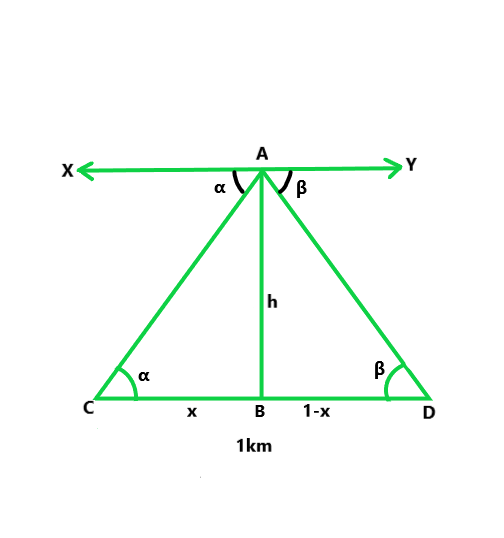XY || CD

Hence ∠C = α, ∠D = β

Let BC = x km then BD = (1 – x) km

Now in △ACB,

tanα = AB/BC = h/x

x = h/tanα

Similarly, in △ABD,

tanβ = AB/BD = h/(1 – x)

h = tanβ – x tanβ

= tanβ – h/tanα x tanβ

h = (tanβ tanα) / (tanα + tanβ)

Hence proved

Question 69. PQ is a post of a given height a and AB is a tower at some distance. If a and p are the angles of elevation of B, the top of the tower, at P and Q respectively. Find the height of the tower and its distance from the post.

Solution:

Let us assume PQ is posted and AB is the tower

Now, the angles of elevation of B, from P and Q are α and β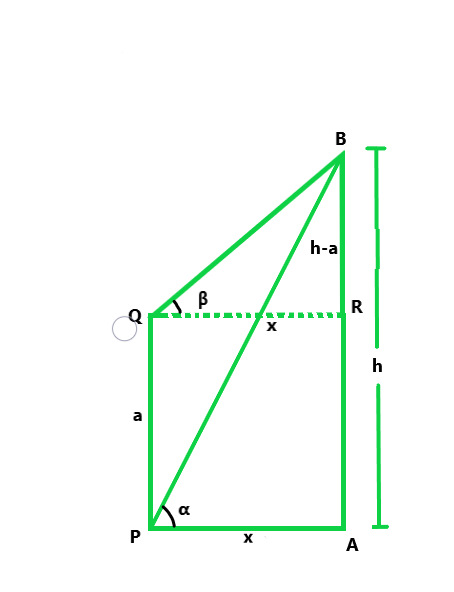PQ = α

Let us assume AB = h and distance between the tower and the post = x

PR || QA

Hence, RA = α and BR = h – α

Now in △ABQ,

tanα = BA/QA = h/x

h = a tanα    ……(i)

Similarly, in △BPR,

tanβ = BR/PR = (h – α) / x

x tanβ = h – α          ……(ii)

From eq(i) and (ii), we get

x(tanα – tanβ) = α

x = α / (tanα – tanβ)

and h = x tanα = α / (tanα – tanβ) x tanα

= α tanα / (tanα – tanβ)

Hence height of tower is α tanα / (tanα – tanβ) and distance b/w them is α / (tanα – tanβ)

Question 70. A ladder rests against a wail at an angle to the horizontal. Its foot is pulled away from the wall through a distance so that it slides a distance b down the wall making an angle p with the horizontal. Show that a / b  = cosα − cosβ / sinβ − sinα

Solution: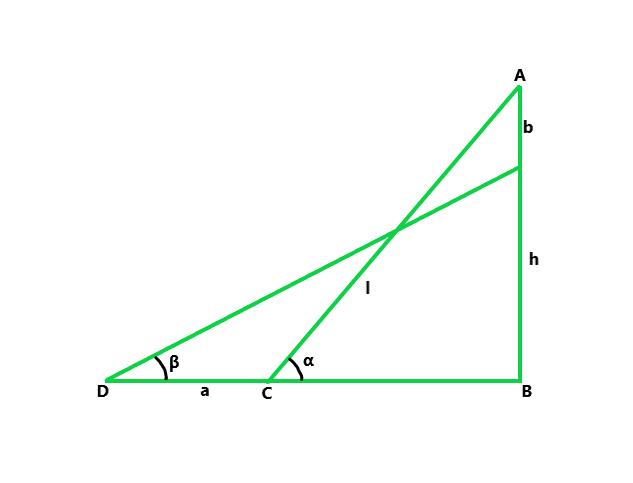From the figure we conclude that

AC and ED is the same stair, so AC = ED

Now cosα = CB/AC

Similarly, cosβ = DB/ED = (α + CB)/AC

sinα = AB/AC = b + EB / AC

sinβ = EB/ED = EB/AC

Now we solve RHS = cosα − cosβ / sinβ − sinα

= (CB/AC – ((α + CB)/AC)) / (EB/AC – (b + EB / AC))

= (CB – a – CB / EB – b – EB)

= a/b

LHS = RHS

Hence proved

Question 71. A tower subtends an angle at point A in the plane of its base and the angle of depression of the foot of the tower at a point b meters just above A is p. Prove that the height of the tower is b tan α cot β.

Solution:

Let us considered TR is the tower that subtends angle α at a point A on the same plane

Given that, AB = b and angle of depression of R from B is β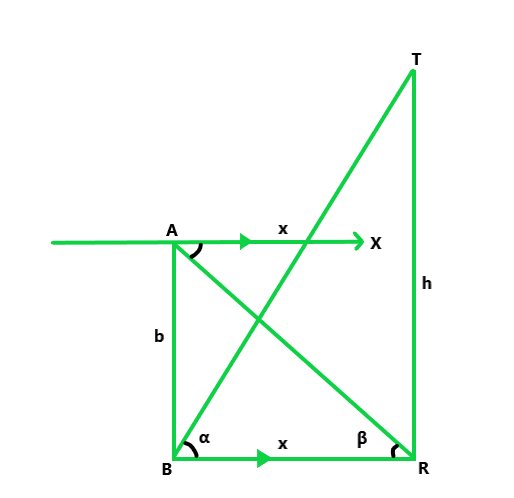BX || AR

Hence, ∠ARB = ∠XBR = β

Let us assume the height of tower TR = h

and AR = x

Now in △ATR,

tanα = h/x

x = h/tanα      …….(i)

Similarly, in △ABR,

tanβ = AB/AR = b/x

x = b/tanβ  …….(ii)

From eq(i) and (ii), we get

h/tanα = b/tanβ

h = b tanα cotβ

Hence, height of the tower is b tanα cotβ.

Hence proved

Question 72. An observer, 1.5 m tall, is 28.5 m away from a tower 30 m high. Determine the angle of elevation of the top of the tower from his eye.

Solution:

Let us assume TR is the tower and CD is the observer

Given that CD is 28.5 m away from the tower TR

Height of the tower TR = 30 m

and height of observer CD = 1.5 m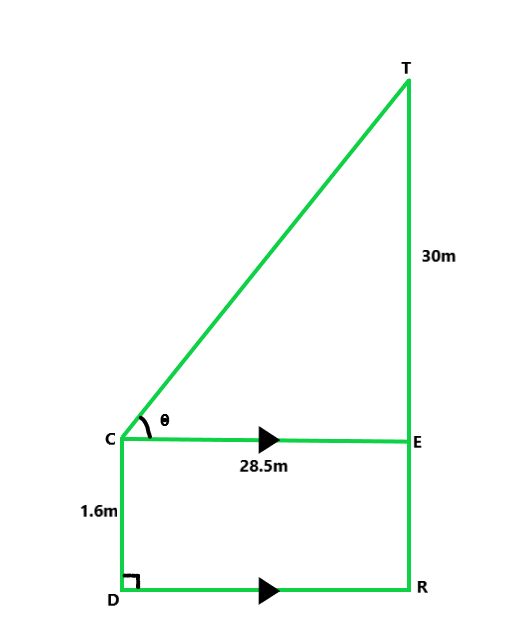Let us assume θ be the angle of elevation of the top of the tower

from the eye of the observer CD,

Now, draw CE || DR

Hence, CE = DR = 28.5 m and ER = CD = 1.5 m

Hence, TE = 30 – 1.5 = 28.5 m

Now in △TCE,

tanθ – TE/CE = 28.5/28.5 = 1 = tan 45°

Hence, the angle of elevation of the top of the tower from his eye is 45°.

Question 73. A carpenter makes stools for electricians with a square top of the side 0.5 m and at a height of 1.5 m above the ground. Also, each leg is inclined at an angle of 60° to the ground. Find the length of each leg and also the lengths of two steps to be put at equal distances.

Solution:

Let us assume AC be the leg of stool whose top is a square-shaped of side AB

Given that, AB = 0.5 m

Also, the height of stool AL = 1.5 m, and angle of inclination by the leg of the stool = 60°Let us assume AC = x m

Now in △ACL,

sin 60° = 1.5/x

√3/2 = 3/2x

x = 1.732 m

Hence, the length of leg = 1.732 m

There are two steps on equal distance,

Distance between two steps = 1.5/3 = 0.5 m

From the given figure

RS || PQ || CD

Hence ∠R = ∠P = ∠C = 60

In △APM,

tan 60° = AN/RN = √3 = 0.5/RN

RN = 0.288 m

Hence, RS = 0.5 + 0.2886 + 0.2886 = 1.077 m.

Question 74. A boy is standing on the ground and flying a kite with 100 m of string at an elevation of 30°. Another boy is standing on the roof of a 10 m high building and is flying his kite at an elevation of 45°. Both the boys are on opposite sides of both the kites. Find the length of the string that the second boy must have so that the two kites meet.

Solution:

Let us considered K be the kite, A and B are two boys flying kites.

Given that Boy B is standing on a building 10 m high and the string AK of the kite of boy A is 100 m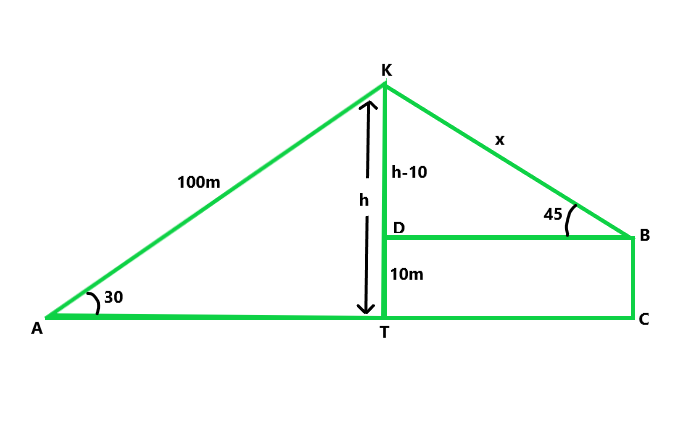Now let us assume h be the height of the kite and x is the length of the string of kite of second boy B

Then, KD = (h – 10) m

Now in △AKT,

sin 30° = h/100 = 1/2 = h/100

h = 50 m

Similarly, in △KDB,

sin 45° = KD/KB = 1/√2 = 40/x

x = 45.6560

Hence, the length of a string of the second kite is 40√2 or 45.656 m.

Question 75. From the top of a lighthouse, the angles of depression of two ships on the opposite sides of it are observed to be α and β. If the height of the lighthouse be h meters and the line joining the ships passes through the foot of the lighthouse, show that the distance between the ship is h(tanα + tanβ) / tanαtanβ meters.

Solution:

Let us assume LH be the lighthouse and A and B are two ships that make

angles of elevation with L are α and β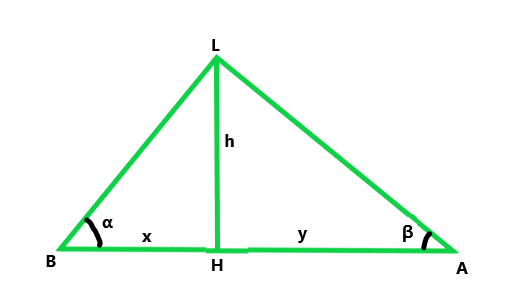Height of lighthouse = h m

Let us assume AH = x and BH = y

Now in △LAH,

tanα = LH/AH = h/x

x = h/tanα   …..(i)

Similarly, in △LBH,

tanβ = LH/HB = h/y

y = h/tanβ    ……(ii)

AB = x + y = h/tanα + h/tanβ

h(1/tanα + 1/tanβ) m

AB = h(tanα + tanβ) / tanα tanβ m.

Hence proved

Question 76. From the top of a tower h meter high, the angles of depression of two objects, which are in the line with the foot of the tower are a and β (β > α). Find the distance between the two objects.

Solution: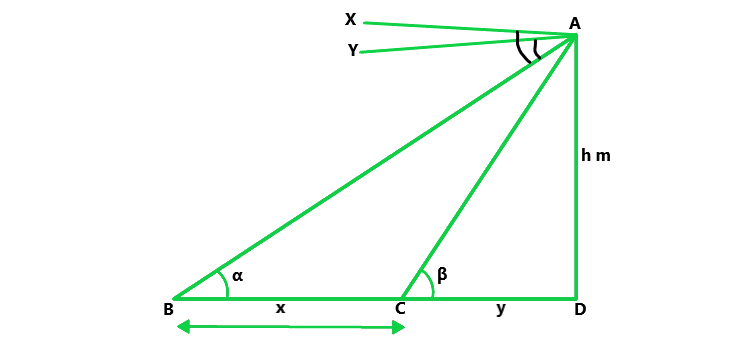Let us considered the distance between two objects is x m and CD = y m

Given that, ∠BAX = α = ∠ABD                              [alternate angle]

∠CAY = β = ∠ACD                                                   [alternate angle]

and the height of tower, AD = h m

Now, in ΔACD,

y = h/tanβ       …..(i)

In △ABD,

x + y = h/tanα

y = h/tanα – x        …..(ii)

From eq(i) and (ii), we get

h/tanβ = h/tanα – x

x = h/tanα – h/tanβ

= h(1/tanα – 1/tanβ) = h(cotα – cotβ)

Question 77. A window of a house is h meter above the ground. From the window, the angles of elevation and depression of the top and bottom of another house situated on the opposite side of the lane are found to be α and β respectively. Prove that the height of the house is h(1 + tan α tan β) meters.

Solution: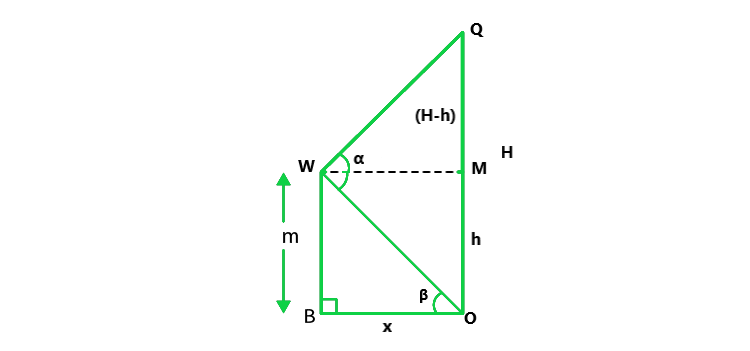Let us considered the height of the other house = OQ = H and OB = MW = x m

Given that, height of the first house = WB = h = MO

and ∠QWM = α, ∠OWM = β = ∠WOB                                 [alternate angle]

Now, in ΔWOB,

tanβ = WB/OB = h/x

x = h/tanβ    ……..(i)

In △QWM,

tanα = QM/WM = OQ – MO / WM

tanα = (H – h)/x

x = (H – h)/tanα           ……..(ii)

From eq(i) and (ii), we get

h/tanβ  = (H – h)/tanα

h tanα = (H – h) tanβ

H = h(tanα + tanβ / tanβ)

= h(1 + tanα.cotβ)

Hence, the required height of the other house is h(1 + tanα.cotβ).

Hence proved

Question 78. The lower window of a house is at a height of 2 m above the ground and its upper window is 4 m vertically above the lower window. At a certain instant, the angles of elevation of a balloon from these windows are observed to be 60° and 30° respectively. Find the height of the balloon above the ground.

Solution:

Let us considered that the height of the balloon from above the ground is H.

A and OP = W2R = W1Q = x

Now, given that, the height of lower window from above the ground = w2P = 2 m = OR

Height of upper window from above the lower window = W1W2 = 4 m = QR

∴ BQ = OB – (QR + RO)

BQ = H – (4 + 2)

BQ = H – 6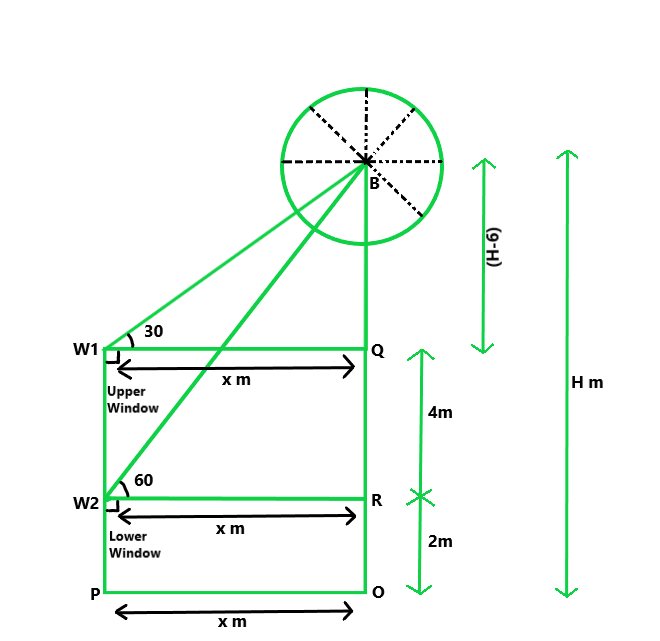and ∠BW1Q = 30°

∠BW2R = 60°

Now in △BW2R,

tan 60° = BR/W2R = (BQ + QR)/x

√3 = (H – 6) + 4 / x

x = (H – 2)/√3  …….(i)

Now in △BW1Q,

tan 30° = BQ/W1Q = (H – 6)/x = 1/√3

x = √3(H – 6)        …….(ii)

From eq (i) and (ii), we get

√3(H – 6) = (H – 2)/√3

Now solving the above equation, we get

H = 8

Hence, the required height of the balloon from above the ground is 8 m.

My Personal Notes arrow_drop_up